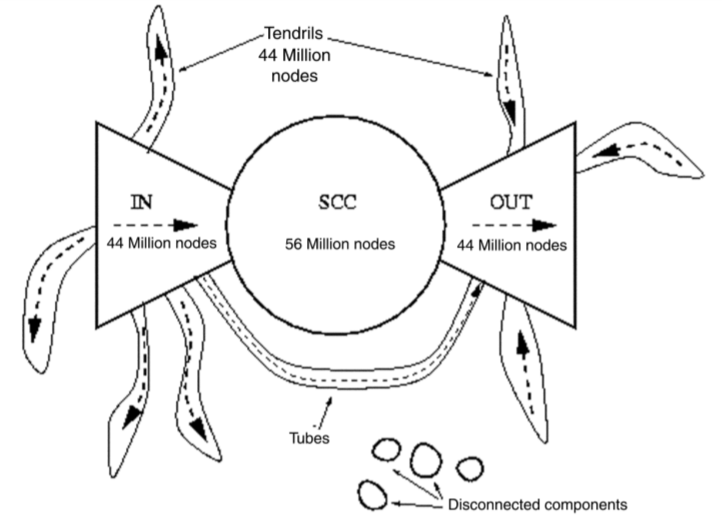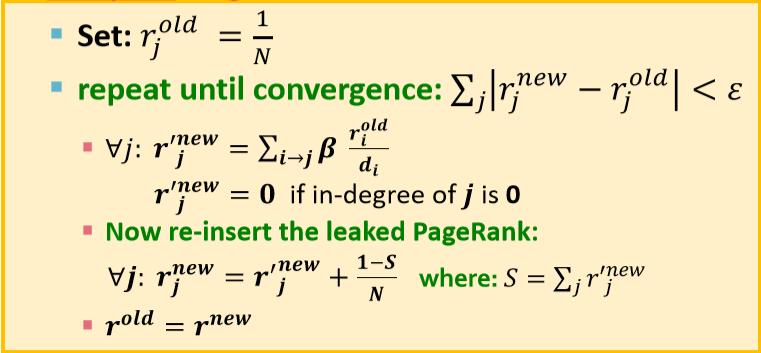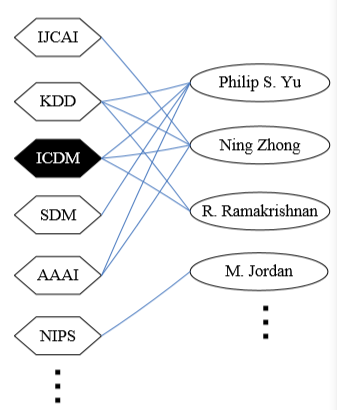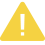# 【图机器学习】cs224w Lecture 10 - PageRank

【摘要】 目录PageRankProblemsPersonalizedPageRank转自本人：https://blog.csdn.net/New2World/article/details/106233258将互联网视为图的话，它必定存在结构上的一些规律。首先回顾一下强连通子图(stronglyconnectedcomponent,SCC)，如果一个有向图的子图内任意节点可以互相到达，...## PageRank

PageRank 的思想很简单，把网页间的链接视为一种均匀的投票机制。比如节点 v 有三个外链，且其自身的初始“票权”为 1。那么迭代开始后，v 会将自己的票平分给三个外链，即每个外链能得到 1/3。同理如果 v 是其它节点的外链，那 v 也会得到其它节点平分后的票。一直这么迭代下去直到收敛就是 PageRank 的核心过程。或者另一种解释是通过 random walk 来理解。如果当前我们位于节点 v，那么我们可以等概率的转移到 v 指向的所有邻接节点。当转移足够多次后会达到一个类似 MDP 中的稳定状态，表示在任意时间点我们位于各个节点的概率。
Talk is cheap, show me the 'math'. $$r$$ 为权重，$$d$$ 为出度。

$r_j = \sum_{i\rightarrow j}\frac{r_i}{d_i}$

Power iteration

1. 初始化：$$r$$
2. 迭代：$$r^{(t+1)}=M\cdot r^{(t)}$$
3. 停止：$$|r^{(t+1)}-r^{(t)}|_1<\epsilon$$

### Problems

1. 遇到 dead ends 时以概率 1 跳转到其它任意节点。
2. 系统判断进入 trap 后，以概率 p 转移到其它任意节点。

$r_j = \sum_{i\rightarrow j}\beta\frac{r_i}{d_i}+(1-\beta)\frac1N$## Personalized PageRank1. PageRank：$$S=[1/N, 1/N, 1/N,..., 1/N]$$
2. Personalized：$$S=[0.1, 0, 0.1,..., 0.2, 0,..., 0.3]$$ (反正和为 1)
3. Random Walk with Restart：$$S=[0, 0, 0, 1, 0,..., 0]$$

【版权声明】本文为华为云社区用户转载文章，如果您发现本社区中有涉嫌抄袭的内容，欢迎发送邮件至：huaweicloud.bbs@huawei.com进行举报，并提供相关证据，一经查实，本社区将立刻删除涉嫌侵权内容。

### 评论 (0)

###### 温馨提示